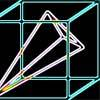#### You may also likeA box of size a cm by b cm by c cm is to be wrapped with a square piece of wrapping paper. Without cutting the paper what is the smallest square this can be?### Plane to See

P is the midpoint of an edge of a cube and Q divides another edge in the ratio 1 to 4. Find the ratio of the volumes of the two pieces of the cube cut by a plane through PQ and a vertex.### Four Points on a Cube

What is the surface area of the tetrahedron with one vertex at O the vertex of a unit cube and the other vertices at the centres of the faces of the cube not containing O?

# Cheese Cutting

##### Age 16 to 18 Challenge Level:

Simplify the problem by making the initial cuts all at right angles so that the large cube is sliced into$8$ smaller cubes.

Align the cube so that the $8$ corners lie at the points $(\pm 1, \pm 1, \pm 1).$

Now consider the plane $x+y+z=0$.

How many of the $8$ smaller cubes does this plane pass through?

What can you say about any cubes that this plane does not pass through? How can you alter this plane to try to get more intersections?

For the second part of the problem don't attempt to work out the theoretical maximum number of pieces as this is exceedingly difficult even for $5$ cuts! You can, however, consider how many pieces each small piece of cheese can be cut into per slice. You can also experiment with various pre-determined 'cutting schemes' to try to find a guaranteed minimum number of possible pieces.